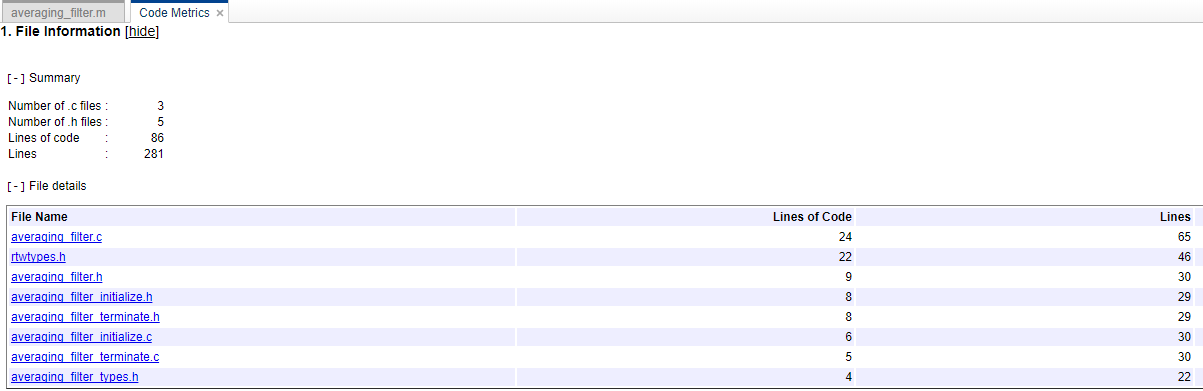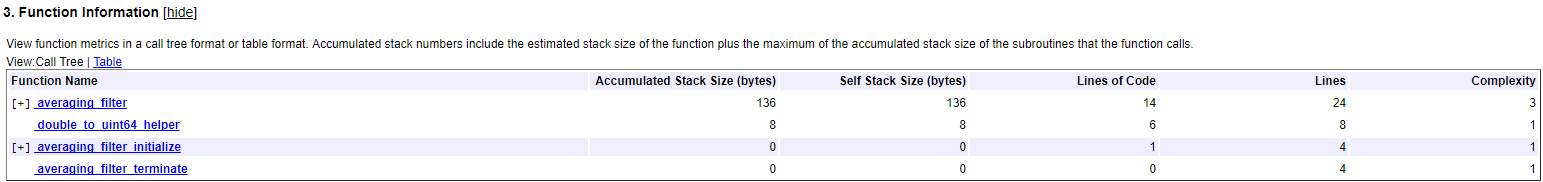Main Content

## Generating a Static Code Metrics Report for Code Generated from MATLAB Code

The static code metrics report contains the results of static analysis of the generated C/C++ code, including generated file information, number of lines, and memory usage. For more information, see Static Code Metrics. To produce a static code metrics report, you must use Embedded Coder® to generate standalone C/C++ code and produce a code generation report. See Code Generation Reports.

By default, static code metrics analysis does not run at code generation time. Instead, if and when you want to run the analysis and view the results, click Code Metrics on the Summary tab of the code generation report.

### Example Static Code Metrics Report

This example runs static code metrics analysis and examines a static code metrics report.

Create the example function `averaging_filter`.

```function y = averaging_filter(x) %#codegen % Use a persistent variable 'buffer' that represents a sliding window of % 16 samples at a time. persistent buffer; if isempty(buffer) buffer = zeros(16,1); end y = zeros(size(x), class(x)); for i = 1:numel(x) % Scroll the buffer buffer(2:end) = buffer(1:end-1); % Add a new sample value to the buffer buffer(1) = x(i); % Compute the current average value of the window and % write result y(i) = sum(buffer)/numel(buffer); end```

Create sample data.

```v = 0:0.00614:2*pi; x = sin(v) + 0.3*rand(1,numel(v));```

Enable production of a code generation report by using a configuration object for standalone code generation (static library, dynamically linked library, or executable program).

```cfg = coder.config('lib', 'ecoder', true); cfg.GenerateReport=true;```

Alternatively, use the `codegen` `-report` option.

Generate code by using `codegen`. Specify the type of the input argument by providing an example input with the `-args` option. Specify the configuration object by using the `-config ` option.

`codegen averaging_filter -config cfg -args {x}`

To open the code generation report, click View report.

To run the static code metrics analysis and view the code metrics report, on the Summary tab of the code generation report, click Code Metrics.

Explore the code metrics report.

1. To see the generated files and the number of lines of code per file, click File Information.2. To see the global variables in the generated code, go to the Global Variables section.To navigate from the report to the source code, click a global variable name.

3. To view the function call tree of the generated code, in the Function Information section, click Call Tree.To navigate from the report to the function code, click a function name.

4. To view the functions in a table format, click Table.The second column, Called By, lists functions that call the function listed in the first column. If multiple functions call that function, all functions are listed. If no functions call that function, this column is empty.

### Requirements for Running Static Code Metrics Analysis After Code Generation

By default, static code metrics analysis does not run at code generation time. Instead, you can run the analysis later by clicking Code Metrics in the code generation report. Running static code metrics analysis after code generation has these requirements and limitations:

• You must have Embedded Coder and use the platform that you used for code generation. Once you run the static code metrics analysis, you can open the code metrics report without Embedded Coder or open it on a different platform.

• If you make the code generation report read-only before you run the analysis, each time that you click Code Metrics, the analysis runs.

### Running Static Code Metrics at Code Generation Time

If you want the code generator to run static code metrics analysis and produce the code metrics report at code generation time:

• In an Embedded Coder code generation configuration object, set `GenerateCodeMetricsReport` to `true`.

• In the MATLAB® Coder™ app, on the Debugging tab, set Static code metrics to `Yes`.

Download guide